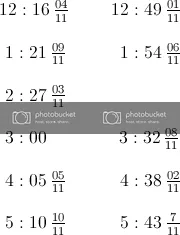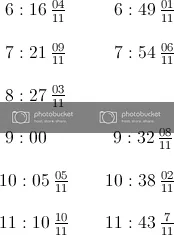# PROBLEM LINK: Champak and Clocks

Author: Yashodhan Agnihotri
Tester: Darshan Lokhande

CAKEWALK

Math

# PROBLEM:

Find the number of times a right angle occurs between the hour hand and the minute hand in the given time range [L,R] , both inclusive, where L and R are hours in the 24-hour format.

# EXPLANATION:

We can easily see that a right angle occurs twice per hour, except the cases of 3 and 9 when it occurs thrice per two hours.
We can iteratively count twice for every hour from L to R and subtract 1 from count for every occurence of 3,9,15,21.
This can also be done by maintaining a prefix array as done in the Tester’s solution below.

Another big corner case is [3,3] , [9,9] , [15,15] , [21,21] for which the answer will be 1 and not 0 as the right angle occurs at that very moment.

# SOLUTIONS:

Setter's Solution
``````#include<bits/stdc++.h>
using namespace std;

int main()
{
ios::sync_with_stdio(false);
cin.tie(0);
int q;
cin >> q;
while (q--)
{
int l, r, i;
cin >> l >> r;
int cnt = 0;
for (int i = l + 1; i < r; i++)
{
if (i == 3 || i == 9 || i == 15 || i == 21)
cnt++;
}
int ans = 2 * (r - l) - cnt;
if (l == r and (r == 3 || r == 9 || r == 15 || r == 21))
ans++;
cout << ans << "\n";
}
return 0;
}
``````
Tester's Solution
``````#include<bits/stdc++.h>
#define boost ios::sync_with_stdio(false); cin.tie(0)
#define ll 				long long int
#define ld 				long double
#define mk 				make_pair
#define pb 				push_back
#define f				first
#define s				second
#define fo(i,a,b) 		for(i=a;i<b;i++)
#define foe(i,a,b) 		for(i=a;i<=b;i++)
#define all(x)			x.begin(),x.end()
// #define vi 				vector<int>
#define vl 				vector <long long int>
// #define pii 			pair <int,int>
#define pll 			pair <long long int, long long int>
// #define vpii			vector< pair<int,int> >
#define vpll 			vector < pair <long long int,long long int> >
#define MOD				1000000007
using namespace std;
const int inf = INT_MAX;
const ll inf64 = LLONG_MAX;

int main()
{
boost;
ll pre;
memset(pre, 0, sizeof pre);
pre = pre = pre = pre = -1;
for (int i = 1; i < 25; i++)pre[i] += pre[i - 1] + 2;
ll t;
cin >> t;
while (t--)
{
ll l, r;
cin >> l >> r;
ll ans;
ans = pre[r] - pre[l];
if (l == 3 or l == 9 or l == 15 or l == 21)ans++;
cout << ans << '\n';
}
return 0;
}
``````

For doubts, please leave them in the comment section, I’ll address them.

4 Likes

But here(when do the minute hand and hour hand be at right angles) I found out something different , if the clock is to be considered in a different way it should have been mentionedwhat do you say about this @zappelectro

1 Like

I didn’t quite grasp what you wanted to be mentioned in the problem. Can you please elaborate ?

just to be sure when do the hands of clock be at right angles according to you @zappelectro
I found out these timesI’m not concerned when they are at right angles. I’m only concerned how many times are they at right angles between [L,R] hours .

Why did you subtract 1 from count for every occurence of 3,9,15,21?
I didn’t understand this thing.Plz Explain me.

Hi, @sam_unlocker, you can easily look up the fact that a right angle occurs twice every hour. But if you check for 2 to 4, then, in the given time range, it only occurs thrice.

1. Around 2:30
2. At exactly 3:00
3. At around 3:35

So, you can clearly see that this is the same case for 9 as well.
Hence, I subtracted 1 from the answer for every occurence of 3,9,15,21 in the range(except endpoints because [L,R] in inclusive).

Sorry for late reply. Hope your doubt is cleared.When it is exactly 3:00, it will be not count in ans? Why? That thing ,I’m not clear…